When you create a computation it is especially important to practice a lot. Writing codes is very unforgiving. One small mistake is enough to stop the computation from working. If you get an error, remember: the code is always right! Stay calm and hold on.

Step 0: creating a computation

Before starting on the actual computation, you need to have a document with questions in it. Remember the names and the values you have given to your questions (or copy them to a notepad). You will need them in the computation.

Do you want to know more about questions? Or do you want tips on how to come up with good names for questions? Take a look at Frequently asked questions of computations.

Go to the left side of the CMS, where it says 'Computation', and click 'create'.Once you have done this, you will see this code: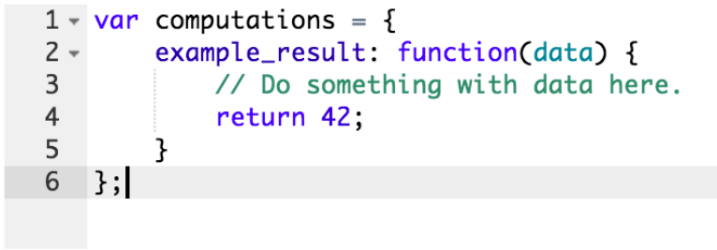This is the standard code you will see when you open a new computation. This is quite handy because the basics of a computation are already there. You just need to change some of the names and add the calculation where it says "do something with data here".

It is handy to name your function something logical. For example 'anxiety' when the function calculates the total score on a questionnaire measuring anxiety. That way you can easily remember it and use it in other places. Keep in mind that the use of capitals or small letters matters, so be consistent in your use of capital and small letters.

In the next example the function is named 'depression'. We open the function body using a curly bracket.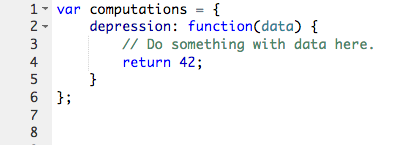You will now write the actual calculation. For this you first have to open a "variable". You can see the variable as a "basket" in which you will collect the data. Open the variable by writing 'var' as the first line within your function. Try to indent like the example so that it is easier to read (or use this online tool for indenting). After 'var', write the name of your variable. It can be different to the name of the function, or the same. Logical names for a variable are for example 'total' or 'sum'.

In the example below, the variable is called "total".Step 3: giving your variable a starting value

We usually assign a starting value of zero to our variable.

The computer does not know what kind of variable it is dealing with. He can see the name, but it is a word. If we set the initial value to 0, the computer knows that the variable is numeric. Only then can he start calculating with it. You can say that he now knows that the "basket" consists of apples, not pears, and that we start with an empty "basket", consisting of 0 apples.

In the example below, the initial value is set to 0.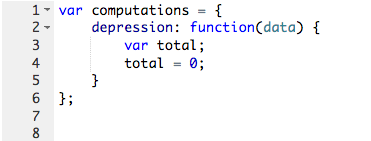We are now going to add the data from a question in your document to the variable 'total'. To refer to a question, you always use 'data.fieldname', or if it is a set or a choices, use 'data.fieldname.option'.

We equal 'total + data.q1;' to our variable. The answer of the question 'q1' is now added to our variable 'total'.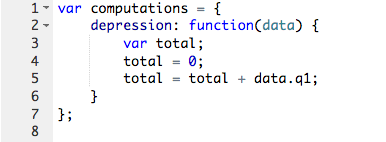Using the apple-metaphore: when you add or substract data-apples, the content of the bucket will change. In this step you let the computer know what to do with the data-apples so that when you get the bucket and the amount of apples back, it will be the outcome of your calculation.

For a simple computation, it is fine to write 'total = total + data.q1'. However, when computations become more complex, it becomes more and more important to be as short and clean as possible. So we advise to use 'total += data.q1' for clarity, although both statements mean the same thing and give the same total score.

 total += data.q1 is the same as total = total + data.q1

Add all the questions you would like to include in your computation to the variable.

In the example the variable consists of the addition of the answers to questions 'q1', 'q2', 'q3'.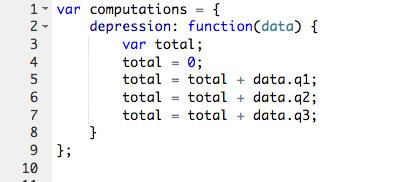Step 6: returning the end value

When you have finished your calculation use the word 'return' and the name of your variable to end the calculation. 'Return' tells the computer to give back the result of your calculation under the name of your function, so you can then use your function name (with the command 'computation:' in front of it) in your session or result document. Close the function body with a curly bracket and then close the entire computation with a curly bracket and a semi-colon.

How do I add another function?

To add another function, put a comma after the curly bracket that closes the previous function. You can then start writing a new function, as shown in the example below.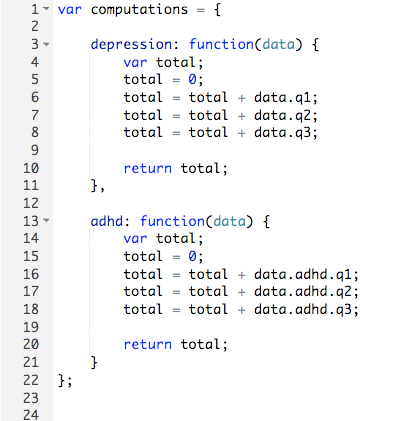We give back the result of the calculation by returning your variable.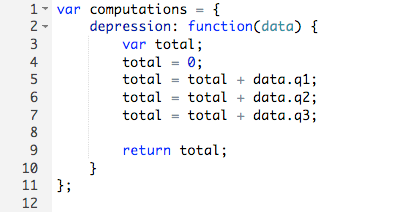Functions, variables, what do you return exactly?

Using the bucket methaphor again, the variable is the bucket with which you work (in which you add or delete apples). You return the final amount of apples/the bucket, over to the function (who then prances around taking all the glory). After returning the variable (like 'total' or 'sum'), the computer ignores it (this is why we can give it such simple, recurring names). In the document or results section we only refer to the function, not the variable (by using for example 'computation:anxiety') in order to retrieve the answer of your computation.Step 7: saving the computation

Click save. The moment of truth: will your computation save? If it does, congratulations!

```Oh no, it gives an error! First, know that this is quite common. Before panicking please take the following steps:
Take a deep breath.Check all the opening and closing commas, brackets, colons and dots. The devil is in the details.Try copy-pasting your code here, a great online tool that checks for errors.Ask for help if you get stuck. When you cancel the computation, copy-paste it somewhere else, so you do not lose your code.```